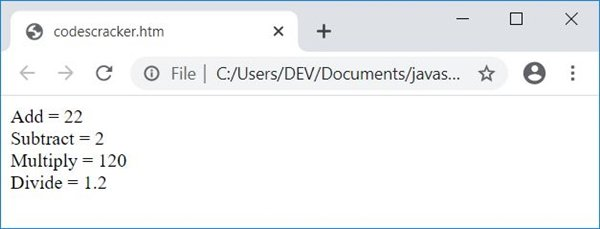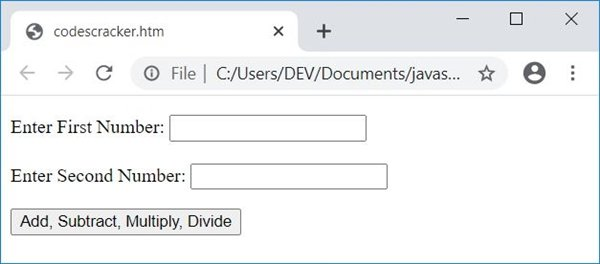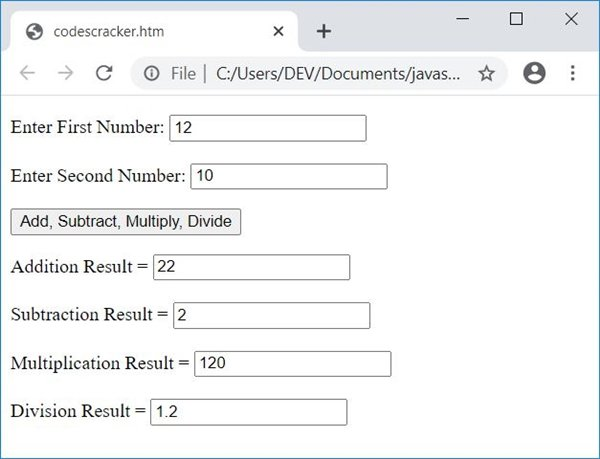# JavaScript Program to Add, Subtract, Multiply, and Divide

In this article, you will learn and get code to apply addition, subtraction, multiplication, and division in JavaScript. There are two programs available here to perform these four famous mathematical operations in JavaScript:

• Add, subtract, multiply, and divide in JavaScript without user input.
• Allow the user to enter the numbers using a textbox.

## Add, subtract, multiply, and divide in JavaScript

The following JavaScript program finds and prints the addition, subtraction, multiplication, and division results of two numbers, say 12 and 10, without getting input from the user.

This is just a demo program showing how these four mathematical operations perform using JavaScript code. Later on, another program for the same task is also given that receives input from the user.

```<!doctype html>
<html>
<body>
<script>
var numOne=12, numTwo=10, res;
res = numOne + numTwo;
document.write("Add = " + res + "<br/>");
res = numOne - numTwo;
document.write("Subtract = " + res + "<br/>");
res = numOne * numTwo;
document.write("Multiply = " + res + "<br/>");
res = numOne/numTwo;
document.write("Divide = " + res + "<br/>");
</script>
</body>
</html>```

Save this code in a file with .html extension. Open the file in a web browser. Here is the output produced by the above code:### Allow the user to enter the number using a textbox

Now this program allows the user to enter two numbers and then perform addition, subtraction, multiplication, and division of the given two numbers.

```<!doctype html>
<html>
<script>
var numOne, numTwo, res, temp;
function fun()
{
numOne = parseInt(document.getElementById("one").value);
numTwo = parseInt(document.getElementById("two").value);
if(numOne && numTwo)
{
temp = document.getElementById("res");
temp.style.display = "block";
res = numOne + numTwo;
res = numOne - numTwo;
document.getElementById("subtract").value = res;
res = numOne * numTwo;
document.getElementById("multiply").value = res;
res = numOne / numTwo;
document.getElementById("divide").value = res;
}
}
</script>
<body>

<p id="input">Enter First Number: <input id="one">
<br/><br/>
Enter Second Number: <input id="two"></p>
<p id="res" style="display:none;">
Subtraction Result = <input id="subtract"><br/><br/>
Multiplication Result = <input id="multiply"><br/><br/>
Division Result = <input id="divide"></p>

</body>
</html>```

Here is its output:Now fill up both boxes with any two numbers, say 12 and 10. Now click on the button Add, Subtract, Multiply, Divide to display the addition, subtraction, multiplication, and division of two entered numbers as shown in the snapshot given below. This is the final output:The following CSS code:

`style="display:none;"`

hides an HTML element. Because it is included in a paragraph whose id is res. So initially this paragraph will be hidden.

When the user clicks on the button Add, Subtract, Multiply, Divide, a function named fun() gets called. And all the statements present inside this function get executed.

The following JavaScript statement:

`numOne = parseInt(document.getElementById("one").value);`

states that the int (integer) value of an HTML element whose id is one gets initialized to the numOne variable. And the following JavaScript statement:

`temp.style.display = "block";`

states that an HTML element whose id is stored in the temp variable gets visible after executing the above JavaScript statement. Here is another JavaScript statement:

`document.getElementById("add").value = res;`

which states that the value of res gets printed in an HTML element whose id is add.

#### Live Output of the Previous Program

Here is the live output produced by the previous JavaScript program on add, subtract, multiply, and divide:

Enter First Number:

Enter Second Number:

JavaScript Online Test

« Previous Program Next Program »